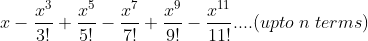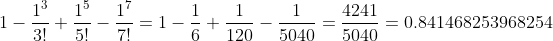# Problem 46828. Approximate functions 2 (★★★★)

Compute the following sum for any given x and number of terms n.Here m! denotes the factorial of m, which the product of all natural numbers upto m, for example
2! = 1x2 = 2
3! = 1x2x3 = 6
5! = 1x2x3x4x5 = 120
If x=1 and n = 4, we haveAvoid using for/while loops.

### Solution Stats

34.62% Correct | 65.38% Incorrect
Last Solution submitted on Jan 28, 2023

### Community Treasure Hunt

Find the treasures in MATLAB Central and discover how the community can help you!

Start Hunting!